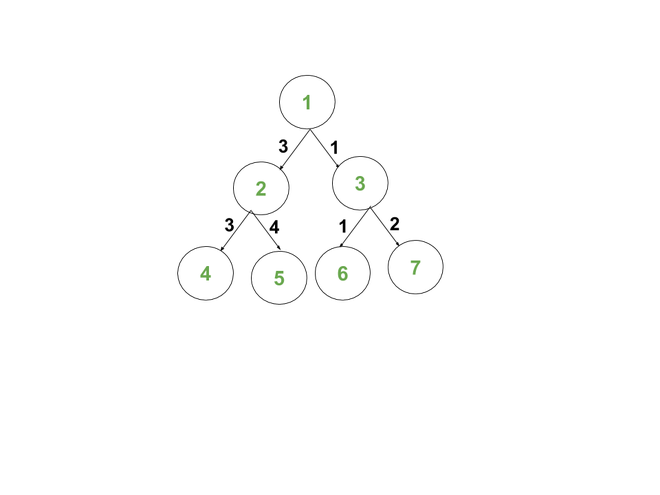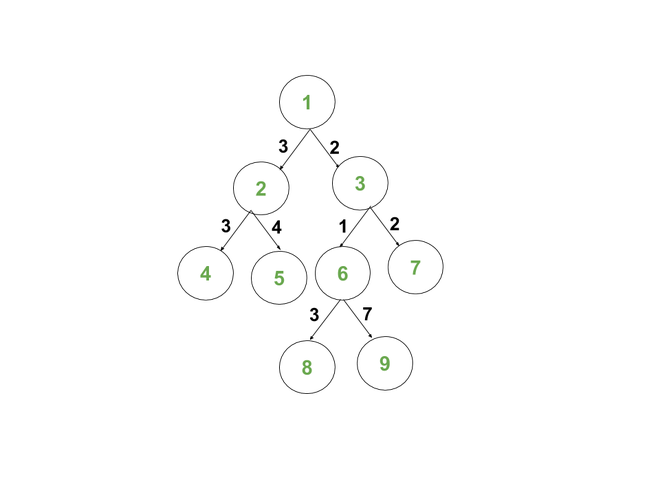# Count nodes having Bitwise XOR of all edges in their path from the root equal to K

• Last Updated : 18 Aug, 2021

Given a Binary Tree consisting of N nodes and two integers R and K. Each edge of the tree has a positive integer associated with it, given in the form {u, v, w} where the edge (u, v) has weight w. The task is to calculate the number of nodes S having Bitwise XOR of all edges in the path from root R to S is equal to K.

Examples:

Input: R = 1, K = 0, N = 7, Edges[][] = {{1, 2, 3}, {1, 3, 1}, {2, 4, 3}, {2, 5, 4}, {3, 6, 1}, {3, 7, 2}}
Output: 2
Explanation:
Representation of the given Binary Tree:The following pair of nodes have a Bitwise XOR of edges in the path connecting them as K = 0:
Pair 1: (1, 4) = (3 ^ 3) = 0
Pair 2: (1, 6) = (1 ^ 1) = 0

Input: R = 1, K = 0, N = 9, Edges[][] = {{1, 2, 3}, {1, 3, 2}, {2, 4, 3}, {2, 5, 4}, {3, 6, 1}, {3, 7, 2}, {6, 8, 3}, {6, 9, 7}}
Output: 3
Explanation:
The representation of given Binary Tree is as follows:The following pair of nodes have a Bitwise XOR of edges in the path connecting them as K = 0:
Pair 1: (1, 4) = (3 ^ 3) = 0
Pair 2: (1, 8) = (2 ^ 1 ^ 3) = 0
Pair 3: (1, 7) = (2 ^ 2) = 0

Approach: The problem can be solved using the Depth First Search approach. Follow the steps below to solve the problem:

1. Initialize the variable ans and xor with 0 to store the number of pairs and the current xor of edges.
2. Traverse the given tree using Depth First Search starting from the given root vertex R.
3. For every node u, visit its adjacent nodes.
4. For each edge {u, v}, if xor is equal to K, increment ans by 1. Otherwise, for the current edge {u, v, w}, update xor as xor = (xor^w) where ^ is the bitwise XOR.
5. After traversing, print the value stored in the counter ans as the number of pairs.

Below is the implementation of the above approach:

## C++

 `// C++ program for the above approach` `#include ` `using` `namespace` `std;`   `// Initialize the adjacency list` `// to represent the tree` `vector > adj;`   `// Marks visited / unvisited vertices` `int` `visited = { 0 };`   `// Stores the required count of nodes` `int` `ans = 0;`   `// DFS to visit each vertex` `void` `dfs(``int` `node, ``int` `xorr, ``int` `k)` `{` `    ``// Mark the current node` `    ``// as visited` `    ``visited[node] = 1;`   `    ``// Update the counter xor is K` `    ``if` `(node != 1 && xorr == k)` `        ``ans++;`   `    ``// Visit adjacent nodes` `    ``for` `(``auto` `x : adj[node]) {`   `        ``if` `(!visited[x.first]) {`   `            ``// Calculate Bitwise XOR of` `            ``// edges in the path` `            ``int` `xorr1 = xorr ^ x.second;`   `            ``// Recursive call to dfs function` `            ``dfs(x.first, xorr1, k);` `        ``}` `    ``}` `}`   `// Function to construct the tree and` `// print required count of nodes` `void` `countNodes(``int` `N, ``int` `K, ``int` `R,` `                ``vector > edges)` `{`   `    ``// Add edges` `    ``for` `(``int` `i = 0; i < N - 1; i++) {` `        ``int` `u = edges[i], v = edges[i],` `            ``w = edges[i];` `        ``adj[u].push_back({ v, w });` `        ``adj[v].push_back({ u, w });` `    ``}`   `    ``dfs(R, 0, K);`   `    ``// Print answer` `    ``cout << ans << ``"\n"``;` `}`   `// Driver Code` `int` `main()` `{` `    ``// Given K and R` `    ``int` `K = 0, R = 1;`   `    ``// Given edges` `    ``vector > edges` `        ``= { { 1, 2, 3 }, { 1, 3, 1 }, ` `            ``{ 2, 4, 3 }, { 2, 5, 4 }, ` `            ``{ 3, 6, 1 }, { 3, 7, 2 } };`   `    ``// Number of vertices` `    ``int` `N = edges.size();`   `    ``// Function call` `    ``countNodes(N, K, R, edges);`   `    ``return` `0;` `}`

## Java

 `// Java program for the ` `// above approach` `import` `java.util.*;` `class` `GFG{`   `static` `class` `pair` `{ ` `  ``int` `first, second; ` `  ``public` `pair(``int` `first, ` `              ``int` `second)  ` `  ``{ ` `    ``this``.first = first; ` `    ``this``.second = second; ` `  ``}    ` `} ` `  `  `// Initialize the adjacency list` `// to represent the tree` `static` `Vector []adj = ` `       ``new` `Vector[``100005``];`   `// Marks visited / unvisited` `// vertices` `static` `int` `visited[] = ` `       ``new` `int``[``100005``];`   `// Stores the required ` `// count of nodes` `static` `int` `ans = ``0``;`   `// DFS to visit each ` `// vertex` `static` `void` `dfs(``int` `node, ` `                ``int` `xorr, ` `                ``int` `k)` `{` `  ``// Mark the current node` `  ``// as visited` `  ``visited[node] = ``1``;`   `  ``// Update the counter ` `  ``// xor is K` `  ``if` `(node != ``1` `&& ` `      ``xorr == k)` `    ``ans++;`   `  ``// Visit adjacent nodes` `  ``for` `(pair x : adj[node]) ` `  ``{` `    ``if` `(visited[x.first] != ``1``) ` `    ``{` `      ``// Calculate Bitwise XOR of` `      ``// edges in the path` `      ``int` `xorr1 = xorr ^ x.second;`   `      ``// Recursive call to dfs` `      ``// function` `      ``dfs(x.first, xorr1, k);` `    ``}` `  ``}` `}`   `// Function to construct the tree and` `// print required count of nodes` `static` `void` `countNodes(``int` `N, ``int` `K,` `                       ``int` `R, ``int``[][] edges)` `{` `  ``// Add edges` `  ``for` `(``int` `i = ``0``; i < N - ``1``; i++)` `  ``{` `    ``int` `u = edges[i][``0``], ` `        ``v = edges[i][``1``],` `    ``w = edges[i][``2``];` `    ``adj[u].add(``new` `pair(v, w ));` `    ``adj[v].add(``new` `pair(u, w ));` `  ``}`   `  ``dfs(R, ``0``, K);`   `  ``// Print answer` `  ``System.out.print(ans + ``"\n"``);` `}`   `// Driver Code` `public` `static` `void` `main(String[] args)` `{` `  ``// Given K and R` `  ``int` `K = ``0``, R = ``1``;` `  `  `  ``for` `(``int` `i = ``0``; i < adj.length; i++)` `    ``adj[i] = ``new` `Vector();` `  ``// Given edges` `  ``int``[][] edges = {{``1``, ``2``, ``3``}, ` `                   ``{``1``, ``3``, ``1``},  ` `                   ``{``2``, ``4``, ``3``}, ` `                   ``{``2``, ``5``, ``4``}, ` `                   ``{``3``, ``6``, ``1``}, ` `                   ``{``3``, ``7``, ``2``}};`   `  ``// Number of vertices` `  ``int` `N = edges.length;`   `  ``// Function call` `  ``countNodes(N, K, R, edges);` `}` `}`   `// This code is contributed by 29AjayKumar`

## Python3

 `# Python3 program for the above approach`   `# Initialize the adjacency list` `# to represent the tree` `adj ``=` `[[] ``for` `i ``in` `range``(``100005``)]`   `# Marks visited / unvisited vertices` `visited ``=` `[``0``] ``*` `100005`   `# Stores the required count of nodes` `ans ``=` `0`   `# DFS to visit each vertex` `def` `dfs(node, xorr, k):` `    `  `    ``global` `ans` `    `  `    ``# Mark the current node` `    ``# as visited` `    ``visited[node] ``=` `1`   `    ``# Update the counter xor is K` `    ``if` `(node !``=` `1` `and` `xorr ``=``=` `k):` `        ``ans ``+``=` `1`   `    ``# Visit adjacent nodes` `    ``for` `x ``in` `adj[node]:` `        ``if` `(``not` `visited[x[``0``]]):`   `            ``# Calculate Bitwise XOR of` `            ``# edges in the path` `            ``xorr1 ``=` `xorr ^ x[``1``]`   `            ``# Recursive call to dfs function` `            ``dfs(x[``0``], xorr1, k)`   `# Function to construct the tree and` `# prrequired count of nodes` `def` `countNodes(N, K, R, edges):`   `    ``# Add edges` `    ``for` `i ``in` `range``(N ``-` `1``):` `        ``u ``=` `edges[i][``0``]` `        ``v ``=` `edges[i][``1``]` `        ``w ``=` `edges[i][``2``]` `        `  `        ``adj[u].append([v, w])` `        ``adj[v].append([u, w])`   `    ``dfs(R, ``0``, K)`   `    ``# Print answer` `    ``print``(ans)`   `# Driver Code` `if` `__name__ ``=``=` `'__main__'``:` `    `  `    ``# Given K and R` `    ``K ``=` `0` `    ``R ``=` `1`   `    ``# Given edges` `    ``edges ``=` `[ [ ``1``, ``2``, ``3` `],[ ``1``, ``3``, ``1` `],` `              ``[ ``2``, ``4``, ``3` `],[ ``2``, ``5``, ``4` `],` `              ``[ ``3``, ``6``, ``1` `],[ ``3``, ``7``, ``2` `] ]`   `    ``# Number of vertices` `    ``N ``=` `len``(edges)`   `    ``# Function call` `    ``countNodes(N, K, R, edges)`   `# This code is contributed by mohit kumar 29`

## C#

 `// C# program for the ` `// above approach` `using` `System;` `using` `System.Collections.Generic;` `class` `GFG{`   `public` `class` `pair` `{ ` `  ``public` `int` `first, ` `             ``second; ` `  ``public` `pair(``int` `first, ` `              ``int` `second)  ` `  ``{ ` `    ``this``.first = first; ` `    ``this``.second = second; ` `  ``}    ` `} ` `  `  `// Initialize the adjacency list` `// to represent the tree` `static` `List []adj = ` `       ``new` `List;`   `// Marks visited / unvisited` `// vertices` `static` `int` `[]visited = ` `       ``new` `int``;`   `// Stores the required ` `// count of nodes` `static` `int` `ans = 0;`   `// DFS to visit each ` `// vertex` `static` `void` `dfs(``int` `node, ` `                ``int` `xorr, ` `                ``int` `k)` `{` `  ``// Mark the current node` `  ``// as visited` `  ``visited[node] = 1;`   `  ``// Update the counter ` `  ``// xor is K` `  ``if` `(node != 1 && ` `      ``xorr == k)` `    ``ans++;`   `  ``// Visit adjacent nodes` `  ``foreach` `(pair x ``in` `adj[node]) ` `  ``{` `    ``if` `(visited[x.first] != 1) ` `    ``{` `      ``// Calculate Bitwise XOR of` `      ``// edges in the path` `      ``int` `xorr1 = xorr ^ x.second;`   `      ``// Recursive call to dfs` `      ``// function` `      ``dfs(x.first, xorr1, k);` `    ``}` `  ``}` `}`   `// Function to construct the tree and` `// print required count of nodes` `static` `void` `countNodes(``int` `N, ``int` `K,` `                       ``int` `R, ``int``[,] edges)` `{` `  ``// Add edges` `  ``for` `(``int` `i = 0; i < N - 1; i++)` `  ``{` `    ``int` `u = edges[i,0];` `     ``int`   `v = edges[i,1],` `    ``w = edges[i,2];` `    ``adj[u].Add(``new` `pair(v, w ));` `    ``adj[v].Add(``new` `pair(u, w ));` `  ``}`   `  ``dfs(R, 0, K);`   `  ``// Print answer` `  ``Console.Write(ans + ``"\n"``);` `}`   `// Driver Code` `public` `static` `void` `Main(String[] args)` `{` `  ``// Given K and R` `  ``int` `K = 0, R = 1;` `  `  `  ``for` `(``int` `i = 0; i < adj.Length; i++)` `    ``adj[i] = ``new` `List();` `  `  `  ``// Given edges` `  ``int``[,] edges = {{1, 2, 3}, ` `                   ``{1, 3, 1},  ` `                   ``{2, 4, 3}, ` `                   ``{2, 5, 4}, ` `                   ``{3, 6, 1}, ` `                   ``{3, 7, 2}};`   `  ``// Number of vertices` `  ``int` `N = edges.GetLength(0);`   `  ``// Function call` `  ``countNodes(N, K, R, edges);` `}` `}`   `// This code is contributed by 29AjayKumar`

## Javascript

 ``

Output:

`2`

Time Complexity: O(N) where N is the number of nodes.
Auxiliary Space: O(N)

My Personal Notes arrow_drop_up
Recommended Articles
Page :